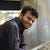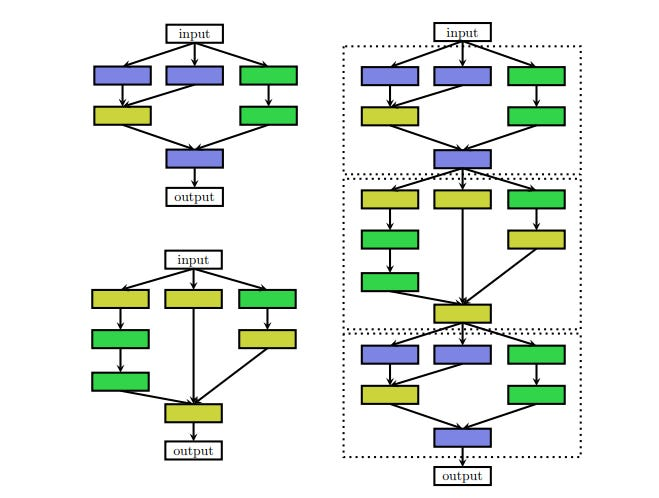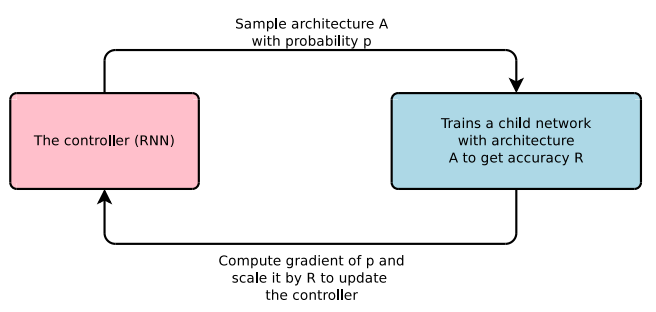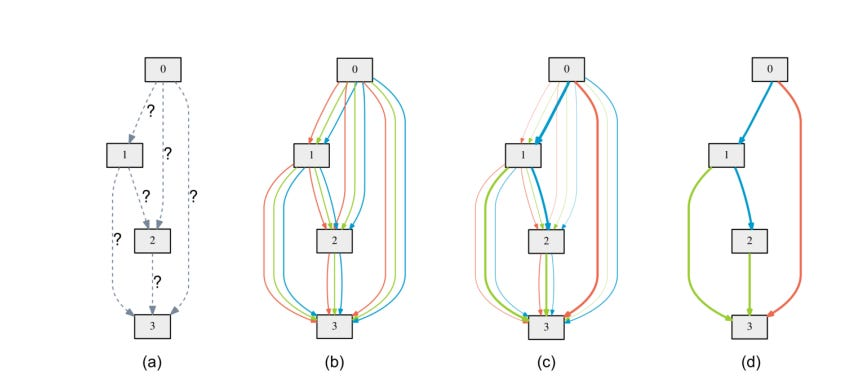# Neural Architecture Search (NAS)- The Future of Deep Learning

Source: Deep Learning on Medium

Most of us probably know about DenseNet. That enormous architecture with skip connections all over. While using this DenseNet as a pre-trained network for my machine learning project, I thought “ How can someone come out with such an architecture? ’’ Soon after I learned that many engineers and scientists with their years of experience build this architecture. And there is more of a hunch rather than complete math that will tell you “ we need to have a 5×5 filter now to achieve the best accuracy ’’. We have wonderful architectures for the image classification task, but many of the young learners like me generally spend hours of time to fix the architecture while working of datasets those are not Image. And we certainly want someone else to do this for us.

That where Neural Architecture Search (NAS), the process of automating architecture engineering comes on the scene. Where we need to just provide a NAS system with a dataset, and it will give us the best architecture for that dataset. NAS can be seen as a subfield of AutoML and has significant overlap with hyperparameter optimization. To understand NAS we need to look deeply what it is doing. It is finding an architecture from all possible architecture by following a search strategy that will maximize the performance. The following figure summarizes NAS algorithm.

It has 3 separate dimensions Search Space, Search Strategy and Performace Estimation.

Search Space defines which neural architectures a NAS approach might discover in principle. It can be chain-like architecture where the output of layer (n-1) is fed as an input of layer (n). Or it can be modern complex architectures with skip connection (multi-branch network).

Some times people do want to use handcrafted outer architecture( macro-architectures) with repeated motifs or cells. In such cases, the outer structure is fixed and NAS only search for the cell architectures. This type of search is known as micro-search or cell search.left: The cell architecture right: cells are put in the handcrafted outer structure(source)

In many NAS methods, both micro and macro structures are searched in a hierarchical fashion; which consists of several levels of motifs. The first level consists of the set of primitive operations, the second level of different motifs that connect primitive operations via a directed acyclic graph, the third level of motifs that encode how to connect second-level motifs, and so on.

To explain the search strategy and performance estimation, three different NAS methods will be discussed in the following part.

Reinforcement Learning

We know about reinforcement learning; where performs some action according to some policy parametrized by θ. Then the agent updates the policy θ from the reward for that action taken. In the case of NAS, the agent produces the model architectures, child network( the action ). Then the model is trained on the dataset and performance of the model on the validation data is taken as a reward.The controller plays the role of an agent and accuracy is taken as the reward

In general Recurrent Neural Network (RNN) is taken as a controller or agent. It produces string and the model is build form that string stochastically.

For example, in picture 5 consecutive RNN output is used to build a filter; starting form filter height to stride width. The output Anchor point is used to indicate skip connections. At layer N, the anchor point will contain N − 1 content-based sigmoids to indicate the previous layers that need to be connected.

The RNN is trained by a policy gradient method to iteratively update the policy θ. The detailed calculation is skipped here which can be found in section 3.2 of the original paper.

Progressive Neural Architecture Search(PNAS)

PNAS does a cell search as discussed in the search space part of this tutorial. They construct cells from blocks and construct the Complete network by adding the cells in a predefined manner.

Cells are connected in a series in a predefined number to form the network. And each cell is formed by several blocks( 5 used in the original paper).

The blocks consist of predefined operations.

The operations have shown the figure are used in the original paper and can be extended.

In the left, an example of a complete is shown. Even in this cell or micro search, there are 10¹⁴ valid combinations to check to find the best cell structure.

So, to reduce complexity first only cells with only 1 blocks are constructed. It is easy because with the mentioned operation there are only 256 of different cells are possible. Then top K best-performing cells are chosen to expand for 2 block cells and it is repeated up to 5 blocks.

But, for a reasonable K, too many 2-block candidates to train. As a solution to this problem, a “cheap” surrogate model that predicts the final performance simply by reading the string(cell is encoded into a string) is trained. The data for this training is collected when the cells are constructed, trained and validated.

For example, we can construct all 256 of single block cells and measure their performance. And train the surrogate model with these data. And then use this model to predict the performance of 2 block cells without actually training and testing them. And of course, the surrogate model should be capable of handling variable size input. And then top K best performing 2 block cells as predicted by the model is chosen. These 2 blocks cells are then actually trained, the “surrogate’’ model is finetuned and these cells are expanded to 3 blocks and it is iterated.

Differentiable Architecture Search(DARTS)

The search space for neural architectures are discrete i.e one architecture is different from the other by at least a layer or some parameter in the layer, for example, 5×5 filter vs 7×7 filter. In this method, a continuous relaxation is applied to this discrete search which to enable direct gradient-based optimization.

The cell we search can be though as a directed acyclic graph where Each node x is a latent representation (e.g. a feature map in convolutional networks) and each directed edge (i, j) is associated with some operation o(i,j) (convolution, max-pooling, etc) that transforms x(i) and stored a latent representation at node x(j)

the output of each node can be calculated by the equation at the left. The nodes are enumerated in such a way, that there is an edge(i,j) from node x(i) to x(j), then i<j.

In continuous relaxation, instead of having a single operation between two nodes. a convex combination of each possible operations is used. To modeled this in graph multiple edges between two nodes are kept, each corresponds to a particular operation. And each edge also has a weight α.

Now O(i,j) the operation between node x(i) and x(j) is a convex combination of a set of operations o(i,j) where o(.)ϵ S, where S is the set of all possible operations.

The output of O(i,j) is calculated by the left equation.

Denote by Lₜᵣₐᵢₙ and Lᵥₐₗ the training and the validation loss, respectively. Both losses are determined not only by the architecture parameters α but also by the weights ‘w’ in the network. The goal for architecture search is to find α∗ that minimizes the validation loss Lᵥₐₗ(w ∗ , α∗ ), where the weights ‘w∗’ associated with the architecture are obtained by minimizing the training loss.

w∗ = argmin_ Lₜᵣₐᵢₙ(w, α∗ ).

This implies a bilevel optimization problem with α as the upper-level variable and w as the lower-level variable:

α * = argmin Lᵥₐₗ(w ∗ (α), α)

s.t. w ∗ (α) = argmin Lₜᵣₐᵢₙ(w, α)

After training some α’s of some edge becomes much larger than the others. To derive the discrete architecture for this continuous model, in between two nodes the only edge with maximum weight is kept.a) Operations on the edges are initially unknown. b) Continuous relaxation of the search space by placing a mixture of candidate operations on each edge c) some weights increases and some falls during bilevel optimization d) final architecture is constructed only by taking edges with the maximum weight between two nodes.

When the cells are found, these are then used to construct larger networks.

The tutorial ends here. Many details are not covered and many methods are not discussed here. NAS has become a very active field and to keep track of the works being done we can follow this AutoML website.